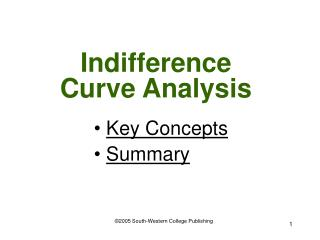# Indifference Curve Analysis - PowerPoint PPT PresentationDownload PresentationIndifference Curve Analysis

Indifference Curve AnalysisDownload Presentation## Indifference Curve Analysis

- - - - - - - - - - - - - - - - - - - - - - - - - - - E N D - - - - - - - - - - - - - - - - - - - - - - - - - - -
##### Presentation Transcript

1. Indifference Curve Analysis • Key Concepts • Summary ©2005 South-Western College Publishing

2. What does this appendix cover? A method for determining equal levels of satisfaction or total utility for different bundles of goods without an exact measure of utils

3. What is an indifference curve? A curve showing the different combinations of two goods that provide the same satisfaction or total utility to a consumer

4. A Consumer’s Indifference Curve Quantity of steak A 20 B 15 C Quantity of lobster 10 D 5 Indifference Curve 4 12 16 8

5. What marginal rate of substitution? The rate at which a consumer is willing to substitute one good for another good without a change in total utility

6. What does the MRS equal? The MRS equals the slope of an indifference curve at any point on the curve

7. What conclusion can we make of indifference curves? The slope of the indifference curve is negative and equal to the marginal rate of substitution (MRS), which declines as one moves downward along the curve

8. What is an indifference map? A selection of indifference curves with each curve representing a different level of satisfaction or total utility

9. What conclusion can we make of indifference maps? Each consumer has a set of indifference curves that form a map

10. A Consumer’s Indifference Map 8 6 Quantity of Steak 4 I 4 I1 I 3 2 I 2 I 1 Q 1 2 3 4 Quantity of Lobster

11. What is a budget line? A line that represents all combinations of two goods that a consumer can purchase with a fixed amount of money given the price of each good

12. A Consumer’s Budget Line 8 6 Quantity of Steak 4 Budget Line 2 Q 1 2 3 4 Quantity of Lobster

13. What does the slope of the budget line equal? The slope of the budget line equals the ratio of the price of good X on the horizontal axis divided by the price of good Y on the vertical axis

14. Slope of budget line Price X Price Y

15. A Consumer’s Indifference Map 8 6 Quantity of Steak 4 I 4 I1 I 3 2 I 2 I 1 Q 1 2 3 4 Quantity of Lobster

16. Deriving a Demand Curve 8 6 Quantity of Steak Y X 4 I 3 2 I 1 Q 1 2 3 4

17. Deriving a Demand Curve \$16 X’ \$12 Y’ \$8 D \$4 1 2 3 4 Quantity of Lobster

18. What conclusion can we draw? Consumer equilibrium occurs where the budget line is tangent to the highest attainable indifference curve. At this unique point, MRS = slope (price ratio of Px/Py)

19. Key Concepts

20. What is an indifference curve? • What is the marginal rate of substitution? • What does the MRS equal? • What is an indifference map? • What is a budget line? • What does the slope of the budget line equal? • What conclusion can we draw?

21. Summary

22. An indifference curve is a curve showing the different combinations of two goods that provide the same satisfaction or total utility to a consumer

23. Our analysis concludes that the slope of the indifference curve is negative and equal to the marginal rate of substitution (MRS), which declines as one moves downward along the curve

24. Consumer equilibrium occurs where the budget line is tangent to the highest attainable indifference curve. At this unique point, MRS = slope (price ratio of Px/Py)

25. END